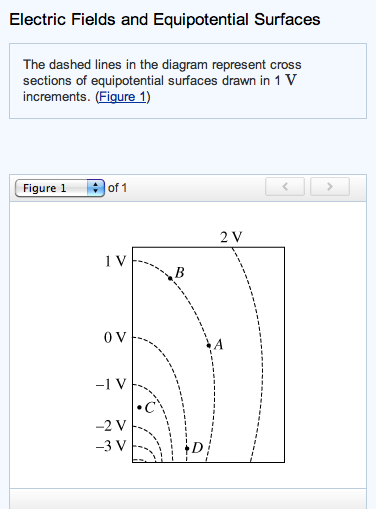# Problem: PART AWhat is the work done by the electric force to move a 1 C charge from A to B?Express your answer in joules.PART BWhat is the work done by the electric force to move a 1 C charge from A to D?Express your answer in joules.Part CThe magnitude of the electric field at point C isA. greater than the magnitude of the electric field at point B.B. less than the magnitude of the electric field at point B.C. equal to the magnitude of the electric field at point B.D. unknown because the value of the electric potential at point C is unknown.

###### FREE Expert Solution

We are required to apply the concepts of work and electric fields.

Work done:

$\overline{){\mathbf{W}}{\mathbf{=}}{\mathbf{-}}{\mathbf{q}}{\mathbf{∆}}{\mathbf{V}}}$

84% (237 ratings)###### Problem Details

PART A

What is the work done by the electric force to move a 1 C charge from A to B?

PART B

What is the work done by the electric force to move a 1 C charge from A to D?

Part C

The magnitude of the electric field at point C is

A. greater than the magnitude of the electric field at point B.

B. less than the magnitude of the electric field at point B.

C. equal to the magnitude of the electric field at point B.

D. unknown because the value of the electric potential at point C is unknown.